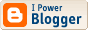<\$BlogRSDURL\$>

The Nacreous Oughts

## A super-Venus around Groombridge 34

(via renderosity)

A planet 5 times the mass of the Earth has been discovered in a sub-habitable orbit around the brighter component of the nearby red dwarf binary Groombridge 34. Both components of the system (now known as Gliese 15, or HIP 475) are flare stars (GX And--with a range of perhaps 0.10 mag-- & GQ And). At a separation of 35" & this distance, the semimajor axis works out to be on the order of 146 AU [125 now] with a period of something like 2600 years [2014 if total masses are 0.48]. Nothing is known about the age of the system.

Hipparcos gives the parallax as 0".28027 & using visual magnitudes of 8.09 & 11.06 i get luminosities of 0.006439420308 & 0.0004176829744. The spectral types are variously given as M1e/M2 & M6/M3.5e. The main article uses T= 3567 K (which is K9 in my tables), R= 0.3863 solar, mass= 0.375 (given elsewhere as 0.414 + 0.08--i would say at least 0.40 for the former). Running some numbers from my own temperature & bolometric correction tables, i find a larger radius for anything cooler than M0.25: BC= -1.1775 T= 3387.5 & 0.019047885, with a derived radius of 0.404595231.

The planetary period of 11.4433 days gives a semimajor axis from 0.072 (M= 0.375) through 0.073 [they give 0.074] (M= 0.40) to 0.076 (M= 0.45). That corresponds to a blackbody temperature of 388, 384, or 375 K. For comparison, Venus is only 328. It would be difficult to avoid a runaway greenhouse, especially as the planet is substantially larger than the Earth.

Just how big? One equation i have, suggests that a rocky planet of 5.35 (min.) Earth masses, would have a radius of 1.56--this corresponds to a density of 1.41 & gravity of 2.3. Since the system is metal-poor (log Fe/H= -0.32), the density might well be less; if as little as 0.575, its radius would be 2.10 & its gravity 1.2. [The system being a metal-poor subdwarf, the anomalously high temperature of the primary sun is somewhat explained.] Log M/H (also given in the main paper) is -0.22 for density 0.684 (Mars is 0.71) is probably a more reasonable lower limit; this gives R= 1.985, & G= 1.35.

Undoubtedly the planet has synchronized its rotation. It does not appear to have been used much in science fiction (apart from video games) but it does have a neighbor less than 2 light years away--Ross 248, which Voyager 2 will pass not far from in 40,000 years; & which appeared in reference books since the 60s as possessing a "ghost planet" with a period of 8 years. That one we might as well call "Xzdjyk" (unless you like "Waughtal's Planet"). For Gliese 15 Ab itself, a case can be made for Tiryns...

(via atlas of the universe)

Labels: